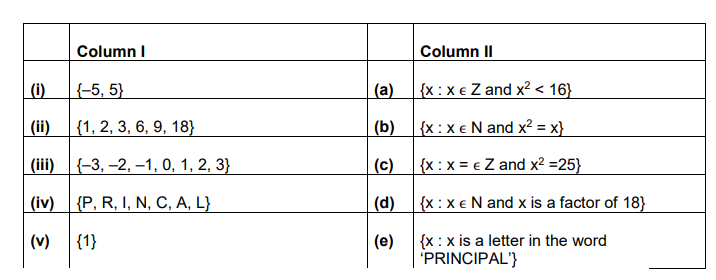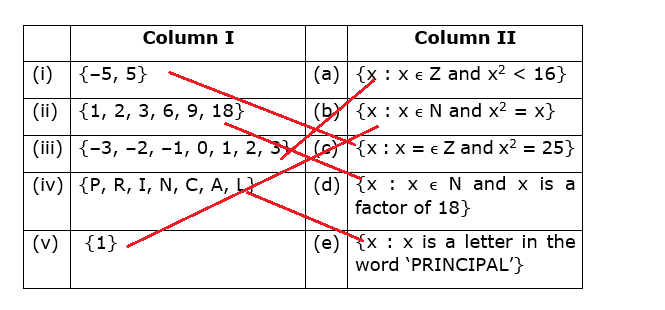# Match each of the sets on the left described in the roster from with the same

Question:

Match each of the sets on the left described in the roster from with the same

set on the right described in the set-builder from:Solution:

(i) {-5, 5}

It can be seen that if we take the square of -5 and 5, the result will be 25

If $x=-5$, then $(-5)^{2}=25$

If $x=5$, then $(5)^{2}=25$

and -5, 5 both are integers

So, $\left\{x: x \in Z\right.$ and $\left.x^{2}=25\right\}$

$\therefore$ (i) matches (c)

(ii) $\{1,2,3,6,9,18\}$

Divisor of 18 are

$18=18 \times 1$

$18=9 \times 2$

$18=6 \times 3$

1, 2, 3, 6, 9, 18 are divisors of 18

So, {x : x ∈ N and x is a factor of 18}

$\therefore$ (ii) matches (d)

(iii) $\{-3,-2,-1,0,1,2,3\}$

$(-3)^{2}=9<16$

$(-2)^{2}=4<16$

$(-1)^{2}=1<16$

$(0)^{2}=0<16$

$(1)^{2}=1<16$

$(2)^{2}=4<16$

$(3)^{2}=9<16$

All are the given elements are integers and satisfying x2 < 16

So, (iii) matches (a)

(iv) $\{\mathrm{P}, \mathrm{R}, \mathrm{I}, \mathrm{N}, \mathrm{C}, \mathrm{A}, \mathrm{L}\}$

There are 9 letters in the word PRINCIPAL out of which P and I are repeated.

So, {x : x is a letter in the word ‘PRINCIPAL’}

$\therefore$ (iv) matches (e)

(v) $\{1\}$

Since, $1 \in N$ and $(1)^{2}=1$

So, $\left\{x: x \in N\right.$ and $\left.x^{2}=x\right\}$

$\therefore$ (v) matches (b)